# aesara.tensor.random.chisquare#

aesara.tensor.random.chisquare = <aesara.tensor.random.basic.ChiSquareRV object>[source]#

A chi square continuous random variable.

The probability density function for chisquare in terms of the number of degrees of freedom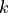is: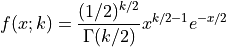for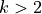.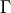is the gamma function: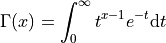This variable is obtained by summing the squaresindependent, standard normally distributed random variables.